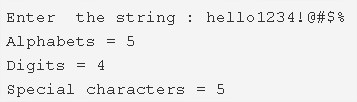# C Program Number Of Alphabets, Digits & Special Character In String | Programs

C program to find the total number of alphabets, digits or special characters in a string – In this article, we will brief in on the multiple methods to find the total number of alphabets, digits or special characters is a string in C programming.

Suitable examples and sample programs have also been added so that you can understand the whole thing very clearly. The compiler has also been added with which you can execute it yourself.

The methods used in this piece are as follows:

• Using Standard Method
• Using Function
• Using Recursion
• Using Pointers and While Loop

A string is nothing but an array of characters. The value of a string is determined by the terminating character. Its value is considered to be 0.Firstly, you need to enter a particular string of your own choice, as per the given example above.

The string that is entered here is as follows:

“hello1234!@#\$%”

As per the title of the blog, we need to find out the number of alphabets, digits and special characters given in the string.

Upon observation, it is seen that:

• Alphabets: 4
• Digits: 4
• Special Characters: 5

Thus, the means used to find out so in C programming are as follows:

## Using Standard Method

1. The ASCII values range of A to Z is 65 to 90, and a to z is 97 to 122 and 0 t0 9 is 48 to 57.

2) Read the entered string and initialize to s.

3) Iterate through string using for loop with the structure for(i=0;s[i];i++)

If the ASCII value of s[i] is in the range between 65 to 90 or 97 to 122 then increase the alphabets count.

If the ASCII value of s[i] is in the range between 48 to 57 then increase the digits count.

If the ASCII value of s[i] is not in the range of the above two conditions then increase the special characters count.

4) Print the alphabets count, digits count and special characters count.

Output:

### Using Function

1. The main() calls the stringcount() function by passing the character array(string) as an argument.

2) The function stringcount() counts the number of alphabets, digits and special characters present in the given string as follows

for loop iterates through the string until s[i] becomes null.

a) Increase the alphabet count if the ASCII value of s[i] is in the range between 65 to 90 or 97 to 122.

b) Increase the digits count if the ASCII value of s[i] is in the range between 48 to 57.

c) If s[i] is not in range of the above two conditions then increase the special characters count.

3) After all iterations of for loop the function prints the values of alphabets count, digits count and special characters count.

Output:

#### Using Recursion

1. The main() calls the stringcount() function, passing the character array(string) as an argument.

2) The function stringcount()

a) if there is no character is available in s[i] i.e s[i] is null then it prints the alphabets count, digits count and special characters count values.

b) If the string contains any characters then,

b.1) If the ASCII value of s[i] is in the range between 65 to 90 or 97 to 122 then increase the alphabets count by 1.

b.2) If the ASCII value of s[i] is in the range between 48 to 57 then digits count will be increased by 1.

b.3) If the ASCII value of s[i] is not in the range of alphabets and digits then increase the special characters count.

b.4) Increase the i value by 1, the function calls itself recursively until s[i] becomes null.

Output:

##### Using Pointers And While Loop
1. Here p is the pointer variable which points the string s.

a) If the first character of the string is null then exit from the while loop i.e while(*p).

b) Otherwise, it checks the ASCII value of the first character. If it is in the range of alphabets then count value of alphabets count will be increased by 1. If the ASCII value is in the range of digits then increase the digits count by 1. If the ASCII value is not in the range of the alphabets and digits then increase the special characters count by 1.

c) Increase the p-value by 1, then p points the next character of the string.

Repeat these three steps a,b,c until there is no character is available at p[i]. Then print the alphabets count, digits count and special characters count.

Output:

x

## C Program To Check If Alphabet, Digit or Special Character | C Programs

C program to check for an alphabet, digit or special character – In this article, ...